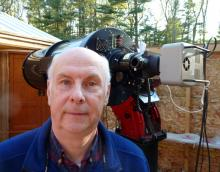# BSM_NM transformation coefficients

2 posts / 0 new
HQABSM_NM transformation coefficients

Here are the transformation coefficients for BSM_NM, from 5 photometric nights using Landolt standards at the beginning of 2016.

Tb_bv = 0.0744

Tv_bv = 0.0075

Tr_vi = 0.0202

Ti_vi = -0.0168

Tbv = 0.9548

Tvi = 1.0157

Arne

MZKRevised Coeffs

Revised Transformation Coeffs generated on 190227 from images from 180224.

description= TG - Version TG_V7.3 beta 5, Telescope= BSM_NM, Time created (UT) = 2019_03_01_02:04:48
[Coefficients]
Tbv= 1.004
Tb_bv= -0.050
Tv_bv= 0.003
Tvr= 1.016
Tv_vr= 0.004
Tr_vr= -0.007
Tri= 1.095
Tr_ri= -0.031
Ti_ri= -0.035
Tvi= 1.043
Tv_vi= 0.016
Ti_vi= -0.031
Tr_vi= -0.017
[Error]
Tbv= 0.030
Tb_bv= 0.074
Tv_bv= 0.044
Tvr= 0.048
Tv_vr= 0.096
Tr_vr= 0.104
Tri= 0.052
Tr_ri= 0.140
Ti_ri= 0.063
Tvi= 0.028
Tv_vi= 0.036
Ti_vi= 0.042
Tr_vi= 0.070
[R Squared Values]
Tbv= 0.925
Tb_bv= 0.015
Tv_bv= 0.011
Tvr= 0.858
Tv_vr= 0.015
Tr_vr= 0.011
Tri= 0.876
Tr_ri= 0.012
Ti_ri= 0.006
Tvi= 0.959
Tv_vi= 0.018
Ti_vi= 0.010
Tr_vi= 0.013BSM_NM 190227 Transform.ini_.txt